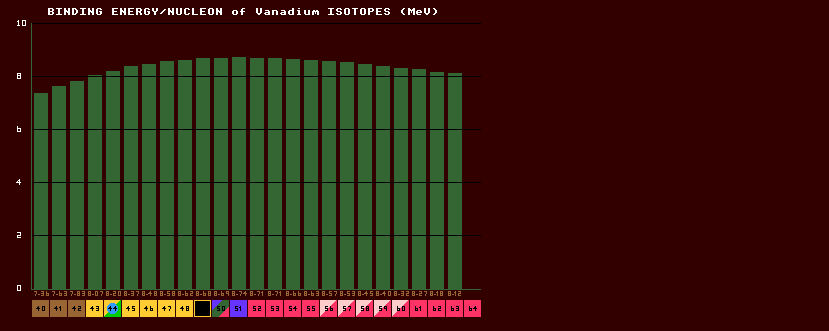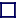### BINDING ENERGY/NUCLEON (BE/N)

(expressed in Mega electron volts, MeV)

 The mass of an isotope is not simply the sum of the masses of all the constituent protons and neutrons within the nucleus, because when they are all gathered together inside the nucleus, some of the mass is lost in binding energy. The BE/N is the total binding energy of the nucleus divided by the number of nucleons within the nucleus. This BE/N is small for elements of low atomic number, but rapidly climbs to a slowly drooping plateau of 8MeV per nucleon. Iron-56 has the highest value, and is thus the most stable element; elements above iron can split into two (as in uranium fission), and elements below iron can combine (fusion, as in the H-bomb); both acts release energy. Shown above is the binding energy per nucleon plot for the isotopes of vanadium. Note that the maximum BE/N occurs near the valley of stability (the inverse beta/beta watershed). For all elements heavier than nitrogen, the BE/N reaches a saturation value of approx 8 MeV per nucleon. Thus the total binding energy is almost proportional to the atomic weight, A, for nuclei with more than 14 nucleons.